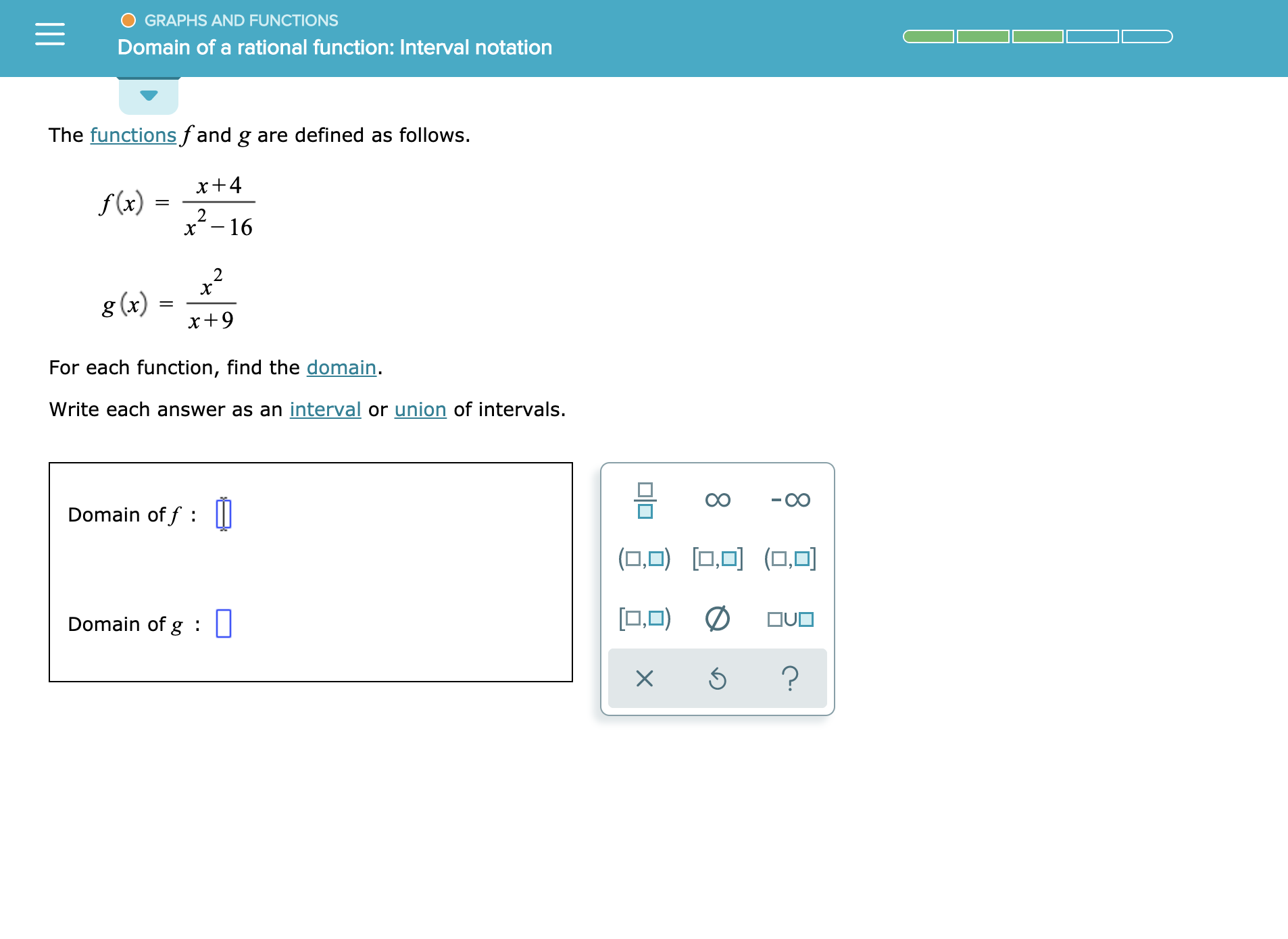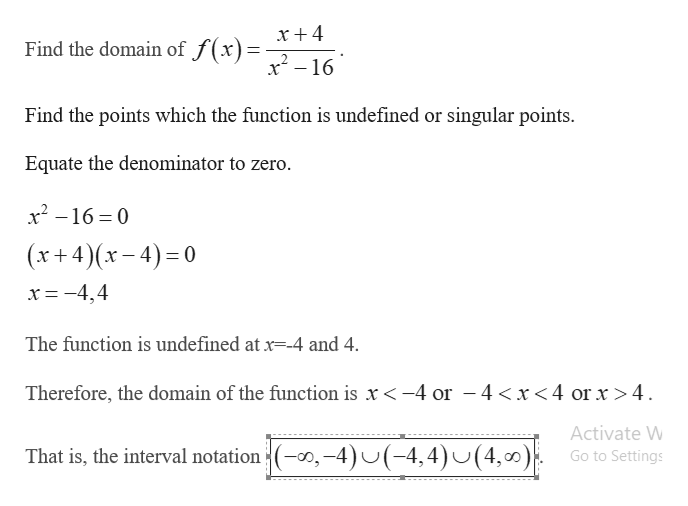GRAPHS AND FUNCTIONSDomain of a rational function: Interval notationThe functionsfand g are defined as follows.x+ 4f(x)=2.x-162g (x)For each function, find the domain.Write each answer as an interval or union of intervals.Domain of f|||(O) (0.ODOUODomain of g : ||?X

Question

See attachedhelp_outlineImage TranscriptioncloseGRAPHS AND FUNCTIONS Domain of a rational function: Interval notation The functionsfand g are defined as follows. x+ 4 f(x) = 2. x-16 2 g (x) For each function, find the domain. Write each answer as an interval or union of intervals. Domain of f ||| (O) (0 .OD OUO Domain of g : || ? X fullscreen
Step 1

The domain of the function is set of all possible values of x where the function is defined.

Step 2

Find the domain of t...help_outlineImage Transcriptionclosex + 4 Find the domain of J (X)x2-16 Find the points which the function is undefined or singular points Equate the denominator to zero. x2-16 0 (x+4)(x-4) 0 x=-4,4 The function is undefined at x--4 and 4 Therefore, the domain of the function is x-4 or -4 4 Activate W (0,-4)(-4,4)J(4,0) That is, the interval notation Go to Settings fullscreen

Want to see the full answer?

See Solution

Want to see this answer and more?

Our solutions are written by experts, many with advanced degrees, and available 24/7

See Solution
Tagged in

Other